PID算法的C程序实现方式，大神带你入门 时间：2018-04-24      来源：未知

1.1比例P

Proportion(比例)，就是输入偏差乘以一个常数。

y=Kp*e(t)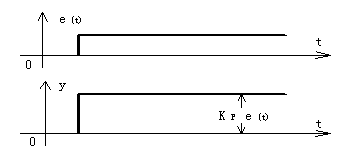1.2 积分I

Integral(积分)，积分作用是指调节器的输出与输入偏差的积分成比例的作用。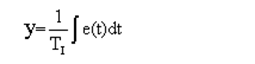Ti是积分时间常数，它表示积分速度的大小，Ti越大，积分速度越慢，积分作用越弱。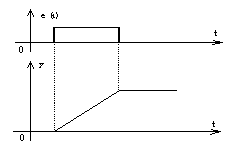1.3 微分D

Derivative(微分)，微分项部分其实就是求电机转速的变化率。也就是前后两次差值的差。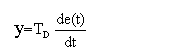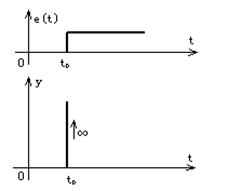2.1 PID算法选择

PID算法中有比例积分调节(PI)，比例微分调节器(PD)，可根据系统要求进行选择，通常为了改善调节品质，往往把比例、积分、微分三种作用组合起来，形成PID调节器。理想的PID微分方程为：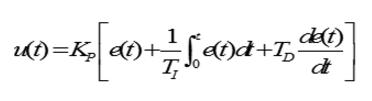e(t) 调节器的偏差信号，它等于给定值与测量值之差

Kp 为比例增益;

T i 积分时间

T d 微分时间

KP /T I 积分系数

KP / T D 微分系数

2.2 PID算法要求

PID需要在一个闭环系统里面(桥黑板)。闭环系统即在控制系统中，有执行处理单元，同时必须有输入反馈单元，电机系统中，必须有编码器、测速电机等测速设备。控制系统原理图如下：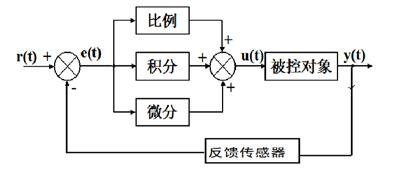2.3 PID参数常用小口诀：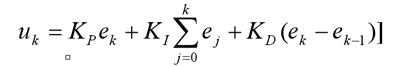k 采样信号，k=0,1,2,…

u k 第k 次采样时刻的计算机输出值

e k 第k 次采样时刻输入的偏差值

e k −1 第k-1 次采样时刻输入的偏差值。

U(k) = P *e(k) + I *[e(k)+e(k-1)+...+e(0)]+ D *[e(k)-e(k-1)]。

u(k) = u(k)-u(k-1) = Kp(e(k) - e(k-1)) + Ki *e(k) + Kd(e(k)) - 2e(k-1) + e(k-2))

C语言理解代码:

//创建变量结构体

struct pid_type{

float Kp; //PI调节的比例常数

float Ti; //PI调节的积分常数

float T; //采样周期

float Ki;

float ek; //偏差e[k]

float ek1; //偏差e[k-1]

float ek2; //偏差e[k-2]

float uk; //u[k]

signed int uk1; //对u[k]四舍五入取整

}pid;

//变量初始

void Pid_Init(void)

{

pid.Kp=4;

pid.Ti=0.005;

pid.T=0.001;

pid.Ki=0.6; //微分系数Kd=KpTd/T。根据实际调节

pid.ek=0;

pid.ek1=0;

pid.ek2=0;

pid.uk=0;

pid.uk1=0;

}

{

if( gabs(ek)<0.1 )

{

}

else

{

pid.uk=pid.Kp*(pid.ek-pid.ek1)+pid.Ki*pid.ek; //计算控制增量

pid.ek1=pid.ek;

pid.uk1=(signed int)pid.uk;

if(pid.uk>0)

{

if(piduk-piduk1>=0.5)

{

pi.uk1=pid.uk1+1;

}

}

if(piduk<0)

{

if(pid.uk1-pid.uk>=0.5)

{

pid.uk1=pid.uk1-1;

}

}

}

}

1. https://blog.csdn.net/u010312937/article/details/53363831#t3《PID控制算法的C语言实现<完整版>》

2. https://blog.csdn.net/qq229596421/article/details/51419813

3. https://blog.csdn.net/msdnwolaile/article/details/51038196